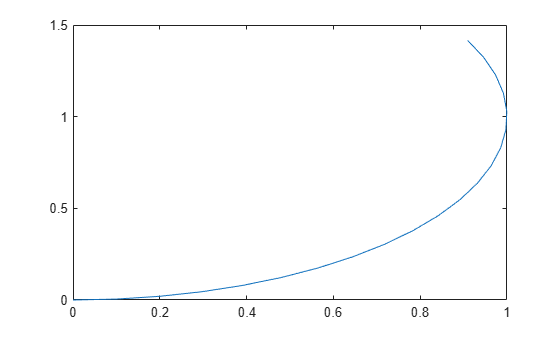# bicycleKinematics

Bicycle vehicle model

## Description

`bicycleKinematics` creates a bicycle vehicle model to simulate simplified car-like vehicle dynamics. This model represents a vehicle with two axles separated by a distance, WheelBase. The state of the vehicle is defined as a three-element vector, [x y theta], with a global xy-position, specified in meters, and a vehicle heading angle, theta, specified in radians. The front wheel can be turned with steering angle psi. The vehicle heading, theta, is defined at the center of the rear axle. To compute the time derivative states of the model, use the `derivative` function with input commands and the current robot state.## Creation

### Syntax

``kinematicModel = bicycleKinematics``
``kinematicModel = bicycleKinematics(Name,Value)``

### Description

example

````kinematicModel = bicycleKinematics` creates a bicycle kinematic model object with default property values.```
````kinematicModel = bicycleKinematics(Name,Value)` sets additional properties to the specified values. You can specify multiple properties in any order.```

## Properties

expand all

The wheel base refers to the distance between the front and rear vehicle axles, specified in meters.

The vehicle speed range is a two-element vector that provides the minimum and maximum vehicle speeds, [MinSpeed MaxSpeed], specified in meters per second.

The maximum steering angle, psi, refers to the maximum angle the vehicle can be steered to the right or left, specified in radians. A value of `pi/2` provides the vehicle with a minimum turning radius of 0. This property is used to validate the user-provided state input.

The minimum vehicle turning radius, specified as a numeric scalar, in meters. The minimum radius is computed using the wheel base and the maximum steering angle.

The `VehicleInputs` property specifies the format of the model input commands when using the `derivative` function. The property has two valid options, specified as a string or character vector:

• `"VehicleSpeedSteeringAngle"` — Vehicle speed and steering angle

• `"VehicleSpeedHeadingRate"` — Vehicle speed and heading angular velocity

## Object Functions

 `derivative` Time derivative of vehicle state

## Examples

collapse all

Create a Robot

Define a robot and set the initial starting position and orientation.

```kinematicModel = bicycleKinematics; initialState = [0 0 0];```

Simulate Robot Motion

Set the timespan of the simulation to 1 s with 0.05 s timesteps and the input commands to 2 m/s and left turn. Simulate the motion of the robot by using the `ode45` solver on the `derivative` function.

```tspan = 0:0.05:1; inputs = [2 pi/4]; %Turn left [t,y] = ode45(@(t,y)derivative(kinematicModel,y,inputs),tspan,initialState);```

Plot path

```figure plot(y(:,1),y(:,2))```Lynch, Kevin M., and Frank C. Park. Modern Robotics: Mechanics, Planning, and Control 1st ed. Cambridge, MA: Cambridge University Press, 2017.

 Corke, Peter I. Robotics, Vision and Control: Fundamental Algorithms in MATLAB. Springer, 2011.